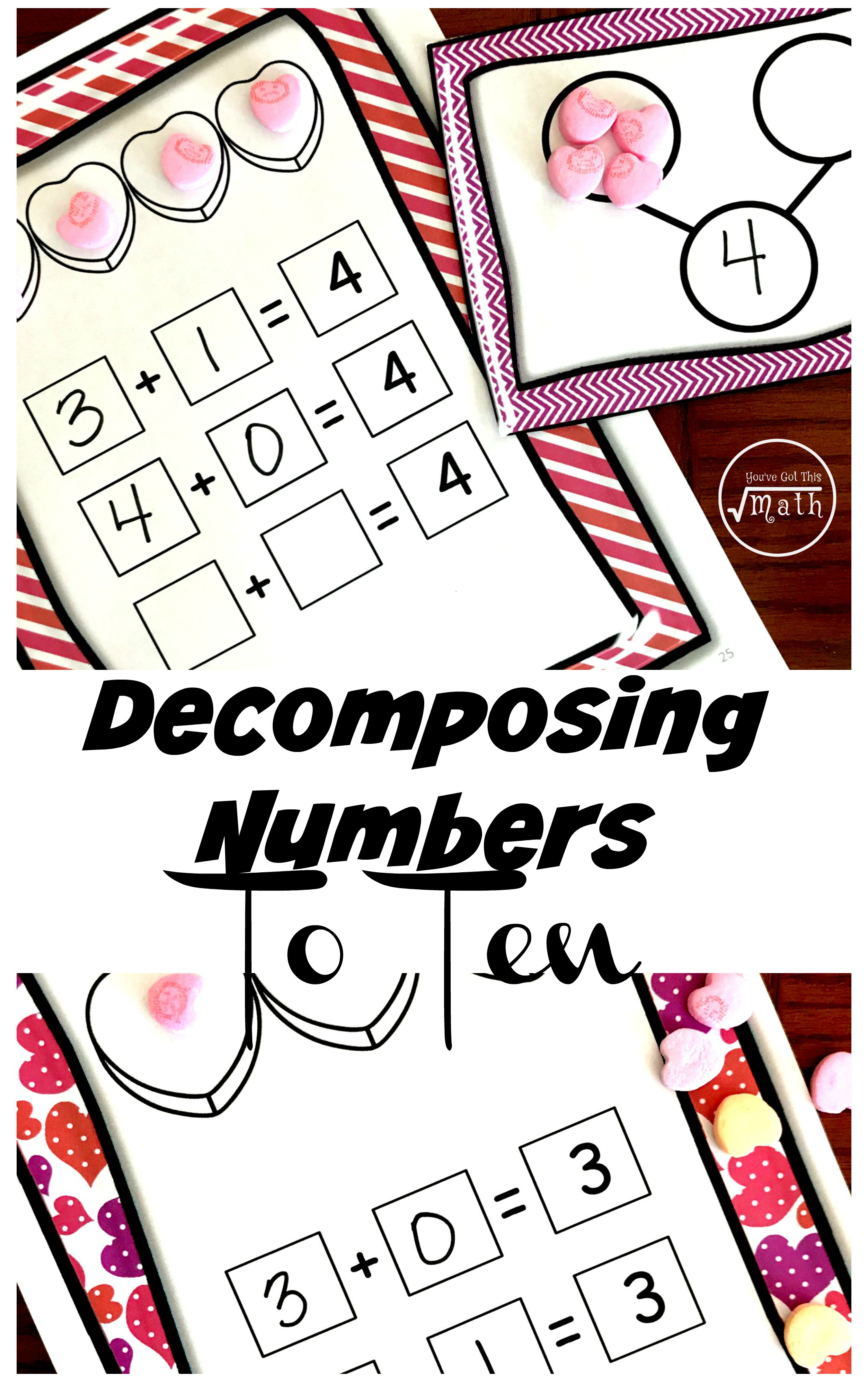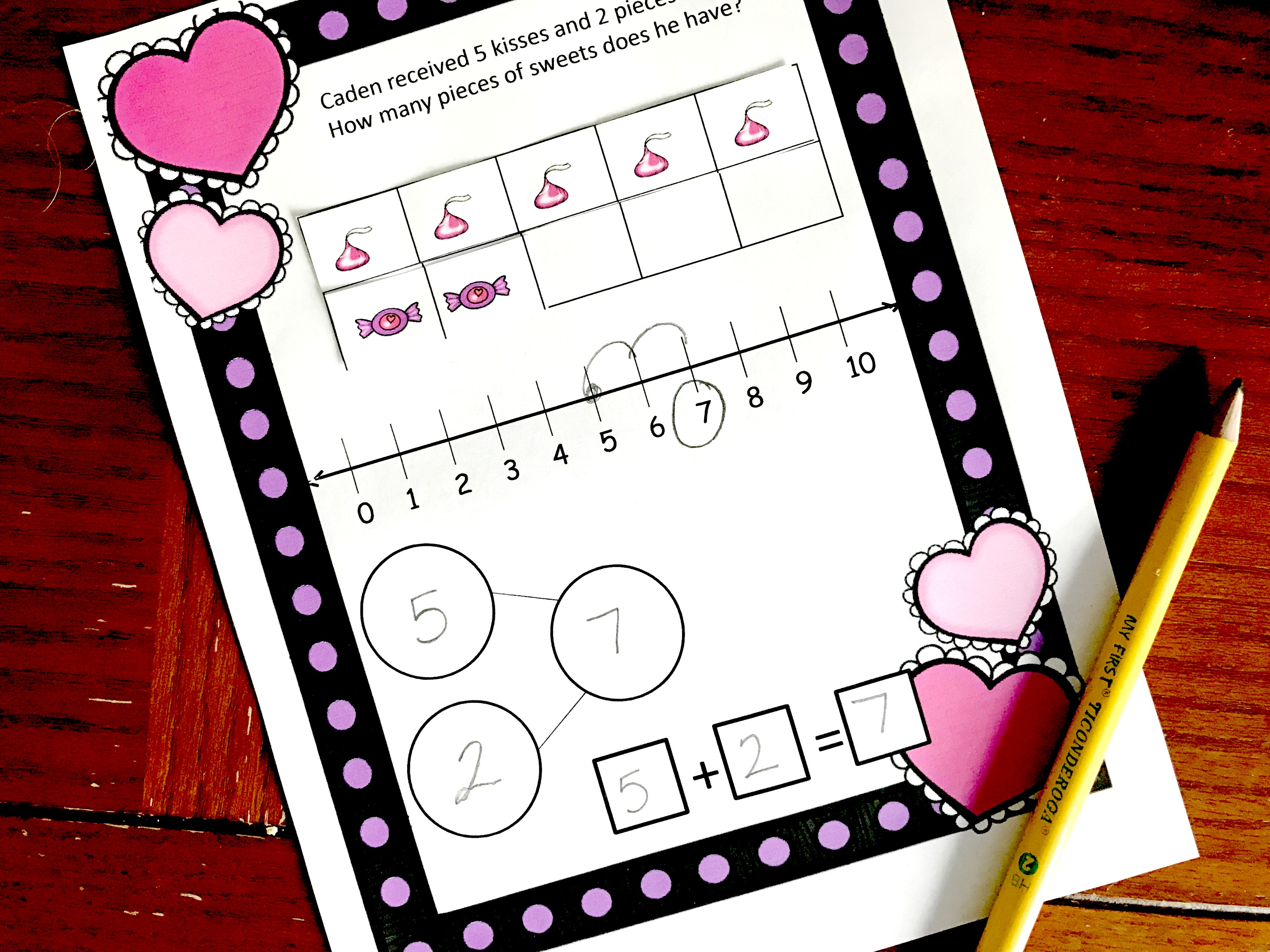Home » Math » Eight FREE Decomposing Numbers to 10 Worksheets

# Eight FREE Decomposing Numbers to 10 Worksheets

Grab your conversation hearts for manipulatives and have some fun with these decomposing numbers to 10 worksheets. By using different colors of the number bond printable, children will figure out the decomposition of numbers less than 10.

Click on the green button at the bottom of the post to get your freebie.This post has affiliate links:

Have you ever done math in your head like this?

20 + 10 = 30 and 5 + 3 = 8 so 25 + 13 = 38

Or have you ever switched a subtraction problem around and thought about it like this….

I know that 5 + 4 = 9 so 9 – 4 = 5. (Yes, that is a little simple for the illustration)

But if you have ever done any math like that in your head…..you are decomposing numbers!! It is an essential part of any math curriculum because it begins to develop number sense. And when that number sense is fully developed children are able to do mental math and have a strong basis for higher level math.

So today, we have a fun activity that will help children focus on decomposing numbers to 10.

## Prep – Work for Decomposing Numbers to 10 Worksheets

• First, print off pages on card stock paper
• Next, place them in sheet protectors or laminate.
• Finally, provide dry erase markers and conversation hearts.

## Directions

The directions for these decomposing numbers to 10 worksheets are pretty easy….and there are two ways for children to work through them.

### Option 1:

One way is to have children only use two colors of hearts. Then they can systematically (I would demonstrate this or do it as a group) figure out the different decompositions of a number.

For example, if I’m working with the number three. We would start by placing three hearts of the same color at the top of the page. Then we would write an equation for that. 3 + 0 = 3Next, we would take away one heart and replace that heart with a different color. Now I have two pinks and one green. My equation is     2 + 1 = 3The decomposing worksheets stop there, but you could discuss that 1 + 2 = 3 too.

### Option 2:

Number bonds are a great way to help children visually see decomposition. And they are easy to use with manipulatives.

All you have to do is count out the number of hearts needed and then work through dividing them up into the circles.

For example, with the number three, we would begin by counting out three hearts and sticking them all in one circle. Now, my kiddos can see that 4 + 0 = 4.

The next step is to take one heart out of one circle and place it in the next circle. Vowlah! 3 + 1 = 4Using the conversation hearts as manipulatives is a wonderful way to develop number senses children as children figure out the decomposition of each number up to 10.

Enjoy!

You’ve Got This

Rachel

This activity gets you working on decomposing teen numbers!or Get These Valentine’s Day Word Problems with Number Bonds